# Try this Unique Approach of Multiplying Two Easy Digits and Obtaining the Answer?!?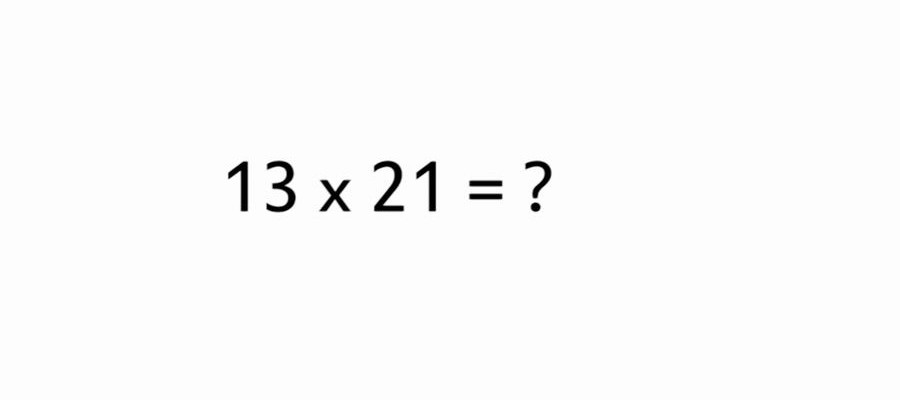When multiplying two digits with each other, it is not necessary to use a calculator or a pencil and paper. Please try the following method.

#### For Example, calculate “13 × 21 =”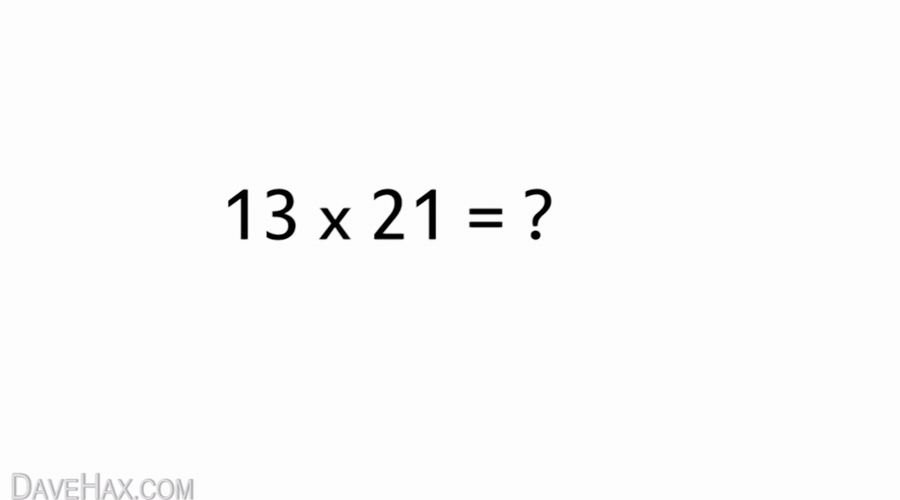#### Draw a Diagonal line by Dividing the “13” to “1” and “3”.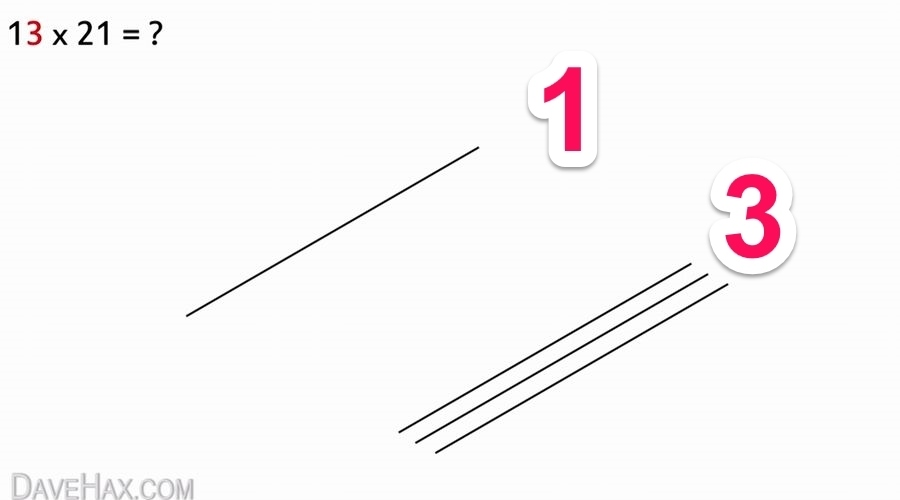#### Furthermore by Dividing the “21” to “2”, “1”

Draw a line so and Intersect the Previous line.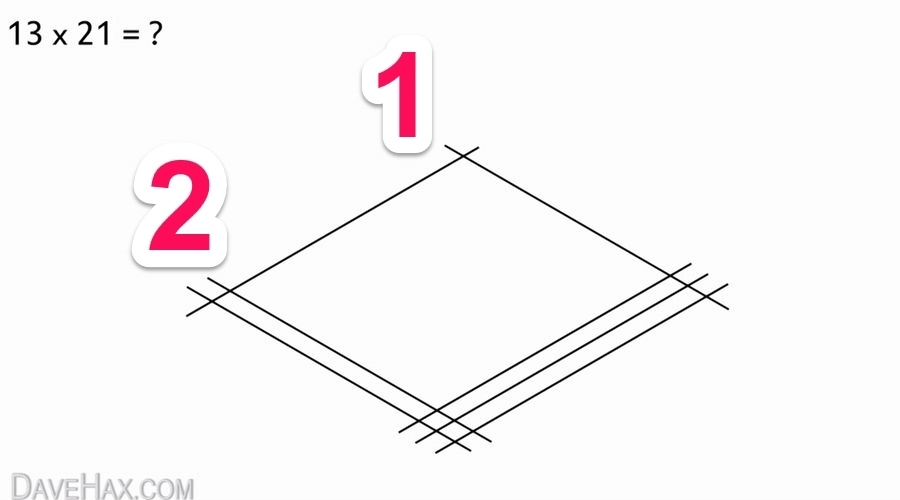#### Count by Dividing the Points of Intersection into Three Areas.

The answer has been derived as “273” in the “2”, “7”, “3”, from left.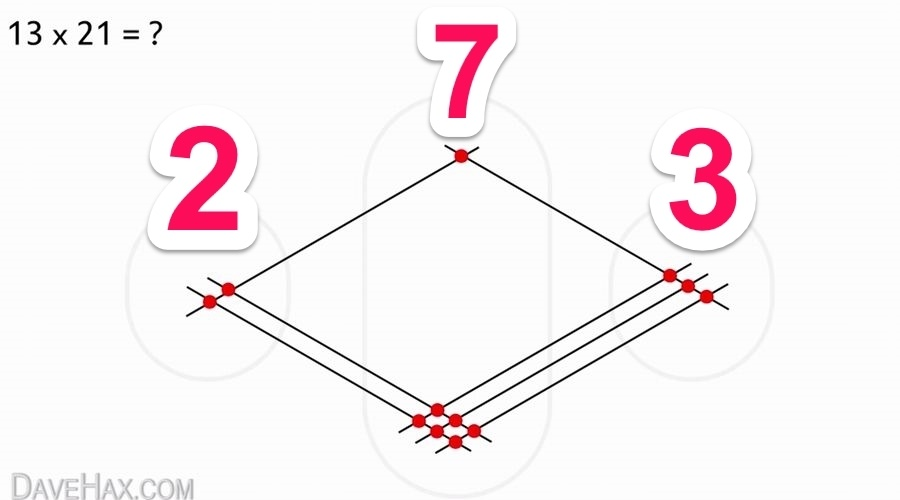See Video Here

※Here is the English Video so You will see and understand the above solution.

I think that the above method is not particularly fast, it was interesting to try this approach instead of the normal calculation process of obtaining the answer.